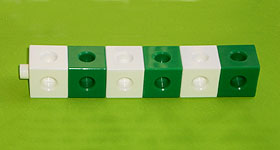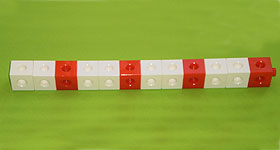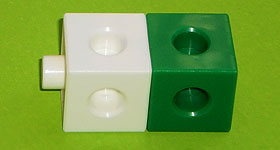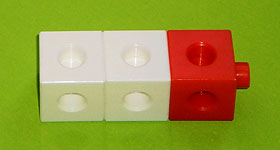Home > Patterns > Misunderstandings > Identical units of repeat

# Identical units of repeat

Repeating patterns can be used to introduce students to many concepts in the early mathematics curriculum, especially multiplication. However, students need to be able to find the unit of repeat in each pattern.

The idea can be introduced using 'trains' of interlocking cubes arranged in a row.A train of six cubes.A train of twelve cubes.

These trains can be made using the following units of repeat.A unit of repeat for the first train.A unit of repeat for the second train.

The first unit is repeated three times, and the second one is repeated four times.

Students can often copy or extend such patterns without being able to find a unit of repeat. For example, students often see the first pattern as 'alternating white and green' rather than 'white-green repeated'.

If students cannot readily identify units of repeat in one-dimensional patterns, then they may not be able to grasp the multiplication that is implicit in such patterns.

## It's harder than you think

This example illustrates the difficulty some children have in finding a unit of repeat for a repeating pattern.

## How do you teach the unit of repeat?

There are many ways to help students learn to identify the unit of repeat.

## Making border patterns

Making a repeating pattern around a border is another way of teaching students the unit of repeat concept.